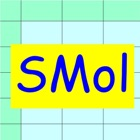Solution Mol Calculator

• SEARCH TYPE

All iPhone applications categories

All iPhone games categories# Solution Mol Calculator

0 0

## Screenshots

Description

Solution Mol calculator is a calculation sheet that solves chemical solution calculation especially solution blending problems.
This software solves the problem not as a black box but as a white box. You need to think the process of solving a problem. This makes your knowledge refresh. The black box solution is convenient, however it has a risk of losing your knowledge. This software has few such risks.

A typical problem is as follows.

(Example)
There is concentrated ammonia (NH3) water. This weight concentration is 28% and density is 0.90 g/cm3. I would like to make ammonia 0.1 molar solution 250 mL by diluting the concentrated ammonia water with pure water. How much liters is the starting concentrated ammonia water?

Solution Mol calculator solves the problem in the following three steps.

//Step 0: Preparation//
Prepare atomic (molecular) weight.
This is as follows.
Molecular weight of NH3 = 17 [g/mol]

//Step 1: Definition//
Enter known data into the SMol sheet.
Rows correspond to solutions. The fifth row is a special row that corresponds to the total blended solution.
Columns correspond to the attribute data of the solution.
First row
Name = 28%NH3, Aw = 17, WgtC = 28, Dty = 0.90
Second row
Name = Water
Fifth row
Name = 0.1molNH3, Aw = 17, MolC = 0.1, SolVol = 250

//Step 2: Calculation//
Attribute data are not independent but mutually related by formulae.
Calculation is a conversion from unknown data to known data using the formulae.
The formulae are embedded in P, G, and V buttons.
Touching out those buttons executes the formulae and changes unknown data to known data.
Touch out buttons as follows.
Set calculation mode to MolC. In this mode, P button calculation is executed by referencing MolC.
Touch out P_SolMol button of the fifth row. W data of the fifth row is changed to known data.
Touch out V_W button of the first row. W data of the first row is changed to known data.
Set calculation mode to WgtC. In this mode, P button calculation is executed by referencing WgtC.
Touch out P_W button of the first row. SolVol data of the first row are changed to known data.
It turns out that the volume of the starting concentrated ammonia water is 1.69 mL.

[Data label]
Name: Solution name (option).
Aw [g/mol]: Atomic or molecular weight of solute.
MolC [mol/mL]: Mol volume concentration of solution.
WgtC [%]: Percent weight concentration of solution.
Dty [g/mL]: Solution density.
SolVol [mL]: Solution volume.
SolWgt [g]: Solution weight.
n [mol]: Mol quantity of solute.
W [g]: Weight of solute.
StdVol [L]: Gas volume of solute at 0 degC and 1 atm.

[Calculation mode]
P button calculation is executed by referencing either WgtC or MolC.
This mode is set by selection of MolC or WgtC.
G button and V button are irrelevant to this calculation mode.

[Buttons and data]
//P button and P data group//
P (put) button inputs data to which the P button is assigned.
P data group is a group of data to which the P buttons are assigned. The P data group has a special character. When one P data is input, all other data of the P data group are updated by formulae.

//G button//
G (get) button gets data from other data using formulae.

//V button//
V (vertically get) button gets data from other row data using the formulae.
The calculation is executed among rows.

//C button//
C (Clear) button clears all row data.

[Color change]
Color change shows what data are input, output, or referenced data in the button execution.
Input data:Blue color
Referenced data:Yellow color
Output data:Green color
Uncalculated data:Red color

[Formulae]
Embedded formulae are as follows.
MolC = 1000 x n / SolVol
WgtC = 100 x W /SolWgt
Dty = W /SplWgt
n = W/Aw
StdVol = 22.414 x n
SolWgt(5) = SolWgt(1) + SolWgt(2) + SolWgt(3) + SolWgt(4)
n(5) = n(1) + n(2) + n(3) + n(4)
W(5) = W(1) + W(2) + W(3) + W(4)